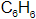×
Get Full Access to Introductory Chemistry - 5 Edition - Chapter 18 - Problem 21q
Get Full Access to Introductory Chemistry - 5 Edition - Chapter 18 - Problem 21q

×

What is the generic structure of alcohols? Write the structures of two specificISBN: 9780321910295 34

Solution for problem 21Q Chapter 18

Introductory Chemistry | 5th Edition

• Textbook Solutions
• 2901 Step-by-step solutions solved by professors and subject experts
• Get 24/7 help from StudySoup virtual teaching assistantsIntroductory Chemistry | 5th Edition

4 5 1 300 Reviews
19
1
Problem 21Q

Describe the structure of benzene. What are the different ways in which this structure is represented?

Step-by-Step Solution:

Step 1 of 2

* Benzene is an organic compound and it is an alkene. And the molecular formula of benzene is.

* It is a colourless compound and highly flammable.

Step 2 of 2

ISBN: 9780321910295

Introductory Chemistry was written by and is associated to the ISBN: 9780321910295. This full solution covers the following key subjects: alcohols, structure, specific, Generic, structures. This expansive textbook survival guide covers 19 chapters, and 2046 solutions. Since the solution to 21Q from 18 chapter was answered, more than 325 students have viewed the full step-by-step answer. This textbook survival guide was created for the textbook: Introductory Chemistry, edition: 5. The full step-by-step solution to problem: 21Q from chapter: 18 was answered by , our top Chemistry solution expert on 05/06/17, 06:45PM. The answer to “?Describe the structure of benzene. What are the different ways in which this structure is represented?” is broken down into a number of easy to follow steps, and 16 words.

Unlock Textbook Solution